# PSAT Math : How to find the whole from the part with percentage

## Example Questions

### Example Question #34 : Percentage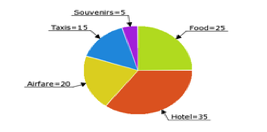David's trip expenses are pictured in the above pie chart (numbers = % of his total expenses). If he spent $75 on taxis, how much did he spend on hotel and souvenirs combined? Possible Answers:$200

$40$250

$175$225

$200 Explanation: David spent$75 on taxis, which were 15% of his total expenses on the trip. He therefore spent 75(100/15) = $500 on the trip altogether. The hotel and souvenirs make up 35% + 5% = 40% of his total expenses. 40% of 500 is$200.

### Example Question #35 : Percentage

30% of what number is 20?

Solve to the nearest hundredth.

66.67

0.67

1.5

150%

66.67

Explanation:

This is a very basic form percentage question. This can be rewritten:

0.3 * x = 20

(Remember, the word "of" in a word problem indicates multiplication, while the word "is" indicates an equals sign).

Solve for x: x = 20 / 0.3 = 66.67

A toy is on sale for 43% off. Its sale price is $21.37. What is the full price? Possible Answers: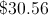None of the available answers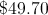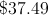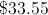Correct answer:Explanation: An algebraic expression for this item is: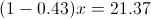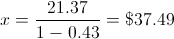### Example Question #37 : Percentage Twenty-six students planned to contribute an equal amount to purchase a gift for their teacher. After 18 students had paid, they had collected$76.50. What is the total price of the gift?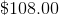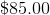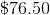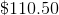If $76.50 had been collected after 18 students had paid, we can determine how much each student contributed:$76.50/18 = $4.25 per student Now we can multiply this by the total number of students (26) to get the full price of the gift: 26 x$4.25 = $110.50 ### Example Question #1 : How To Find The Whole From The Part With Percentage If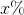ofis, then what isof? Possible Answers: 10 50 200 20 100 Correct answer: 50 Explanation: The first part of the problem tells us that x% of 20 is 50. We can model x% as x/100 or 0.01x. To find x% of 20, we can multiply 0.01x and 20. In other words, we can write the following equation: (0.01x)(20) = 50 Divide both sides by 20. 0.01x = 2.5 Divide both sides by 0.01. x = 250. The question then asks us to find 20% of x. We can represent 20% as 0.2, and we know that x is 250. Therefore, 20% of 250 = 0.2(250) = 50. The answer is 50. ### Example Question #2 : How To Find The Whole From The Part With Percentage Ifof a number is, what isof the number? Possible Answers: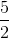Correct answer:Explanation: Let x be the number in question. Then 2/3 * x = 18. x = 18 * 3/2 = 27 Now find 1/9 of 27: 1/9 * 27 = 3 ### Example Question #1 : How To Find The Whole From The Part With Percentage A salesperson for a car dealership earns 16% commission on all sales. She has sold one vehicle this week, a$82,000 SUV on Tuesday, and today is Saturday. She would like to earn a total of $10,000 in commissions by week's end. At the very least, how much in sales must she accomplish today to meet her goal? Possible Answers: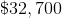She has already met her goal.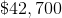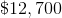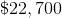Correct answer: She has already met her goal. Explanation: The salesperson has already earned a commission of 16% of$82,000, which is equal to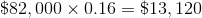.